# Introduction to timbeR

### Fitting taper models

The timbeR package has functions to estimate diameters along the stem, height at which certain diameter values occur and total or partial volumes. For this, it is necessary to fit a taper model that describes the stem profile. This vignette aims to exemplify the regression analysis needed to fit the three models whose the functions mentioned above are implemented in the timbeR package. The possible models to be used are:

• 5th degree polynomial taper function (Schöepfer, 1966) $\frac{d_i}{dbh}=\beta_0\frac{h_i}{h}+\beta_1\left(\frac{h_i}{h}\right)^2+\beta_2\left(\frac{h_i}{h}\right)^3+\beta_3\left(\frac{h_i}{h}\right)^4+\beta_4\left(\frac{h_i}{h}\right)^5$

• Kozak (2004) variable-form taper model $d_i = \beta_0dbh^{\beta_1}\left[\frac{1-\left(\frac{h_i}{ht}\right)^{1/4}}{1-p^{1/4}}\right]^{\beta_2+\beta_3\left(\frac{1}{e^{dbh/ht}}\right)+\beta_4dbh^{\left[\frac{1-\left(\frac{h_i}{ht}\right)^{1/4}}{1-p^{1/4}}\right]}+\beta_5\left[\frac{1-\left(\frac{h_i}{ht}\right)^{1/4}}{1-p^{1/4}}\right]^{dbh/ht}}$

• Bi (2000) trigonometric variable-form taper model $d_i=dbh\left[ \left( \frac{log\;sin \left( \frac{\pi}{2} \frac{h_i}{ht} \right)} {log\;sin \left( \frac{\pi}{2} \frac{1,3}{ht} \right)} \right) ^{\beta_0+\beta_1sin\left(\frac{\pi}{2}\frac{h_i}{ht}\right)+\beta_2cos\left(\frac{3\pi}{2}\frac{h_i}{ht}\right)+\beta_3sin\left(\frac{\pi}{2}\frac{h_i}{ht}\right)/\frac{h_i}{ht}+\beta_4dbh+\beta_5\frac{h_i}{ht}\sqrt{dbh}+\beta_6\frac{h_i}{ht}\sqrt{ht}} \right]$

where:
$$\beta_1,\beta_2,...,\beta_n$$ = model parameters;
$$h_i$$ = height to section i of the stem;
$$d_i$$ = diameter in section i of the stem;
$$dbh$$ = diameter at breast height;
$$h$$ = total height of the tree;
$$p$$ = first inflection point calculated in the segmented model of Max and Burkhart (1976).

We will perform a regression analysis on the tree_scaling dataset, using the aforementioned models. The data can be accessed by importing the timbeR package.

# install.packages("devtools")
# devtools::install_github('sergiocostafh/timbeR')

library(dplyr)
library(timbeR)

glimpse(tree_scaling)
#> Rows: 136
#> Columns: 5
#> $tree_id <dbl> 1, 1, 1, 1, 1, 1, 1, 1, 1, 1, 1, 1, 1, 1, 1, 1, 1, 2, 2, 2, 2,~ #>$ dbh     <dbl> 14.96, 14.96, 14.96, 14.96, 14.96, 14.96, 14.96, 14.96, 14.96,~
#> $h <dbl> 15.61, 15.61, 15.61, 15.61, 15.61, 15.61, 15.61, 15.61, 15.61,~ #>$ hi      <dbl> 0.1561, 0.3122, 0.4683, 0.6244, 0.7805, 1.3000, 1.5610, 2.3415~
#> $di <dbl> 16.55, 15.92, 15.60, 15.18, 14.96, 14.00, 12.73, 12.10, 10.19,~ As we can see, there are five columns in the dataset that refer to the tree id (tree_id), diameter at breast height (dbh), tree total height (h), height at section i (hi) and diameter at hi height (di). A common way to visualize the stem profile from collected data is to plot the relationship between relative diameters and relative heights (di / dbh vs hi / ht), as follows. library(ggplot2) tree_scaling <- tree_scaling %>% mutate(did = di/dbh, hih = hi/h) ggplot(tree_scaling, aes(x = hih, y = did, group = tree_id))+ geom_point()+ labs(x = 'hi / h', y = 'di / dbh')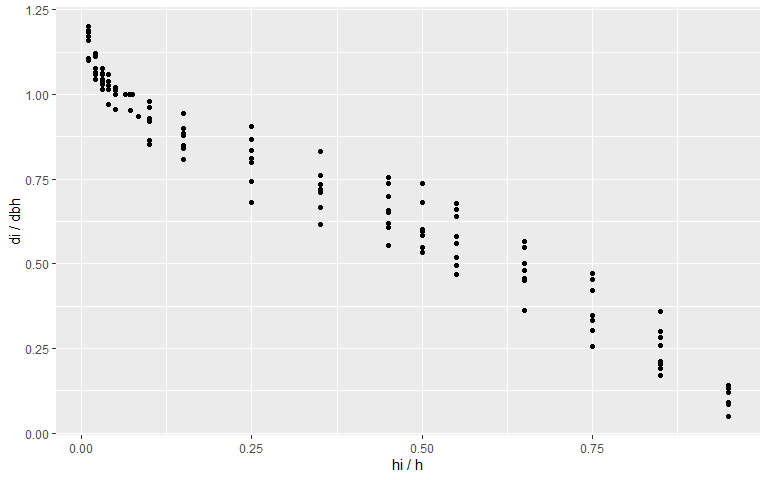Now that we understand the dataset, we can start the regression analysis. The first model we will fit is the 5th degree polynomial. poli5 <- lm(did~hih+I(hih^2)+I(hih^3)+I(hih^4)+I(hih^5),tree_scaling) summary(poli5) #> #> Call: #> lm(formula = did ~ hih + I(hih^2) + I(hih^3) + I(hih^4) + I(hih^5), #> data = tree_scaling) #> #> Residuals: #> Min 1Q Median 3Q Max #> -0.124049 -0.029700 -0.003642 0.032621 0.112321 #> #> Coefficients: #> Estimate Std. Error t value Pr(>|t|) #> (Intercept) 1.15657 0.01615 71.596 < 2e-16 *** #> hih -3.37185 0.46898 -7.190 4.55e-11 *** #> I(hih^2) 13.57792 3.40969 3.982 0.000113 *** #> I(hih^3) -29.92092 9.59285 -3.119 0.002235 ** #> I(hih^4) 29.39935 11.38070 2.583 0.010893 * #> I(hih^5) -10.85327 4.78706 -2.267 0.025028 * #> --- #> Signif. codes: 0 '***' 0.001 '**' 0.01 '*' 0.05 '.' 0.1 ' ' 1 #> #> Residual standard error: 0.05469 on 130 degrees of freedom #> Multiple R-squared: 0.9697, Adjusted R-squared: 0.9685 #> F-statistic: 830.8 on 5 and 130 DF, p-value: < 2.2e-16 tree_scaling <- tree_scaling %>% mutate(di_poli = predict(poli5)*dbh) poli_rmse <- tree_scaling %>% summarise(RMSE = sqrt(sum((di_poli-di)^2)/mean(di_poli))) %>% pull(RMSE) %>% round(2) ggplot(tree_scaling,aes(x=hih))+ geom_point(aes(y = (di_poli-di)/di_poli*100))+ geom_hline(aes(yintercept = 0))+ scale_y_continuous(limits=c(-60,60), breaks = seq(-100,100,20))+ scale_x_continuous(limits=c(0,1))+ labs(x = 'hi / h', y = 'Residuals (%)', title = '5th degree polynomial taper function (Schöepfer, 1966)', subtitle = 'Dispersion of residuals along the stem', caption = paste0('Root Mean Squared Error = ', poli_rmse,'%'))+ theme(plot.title.position = 'plot')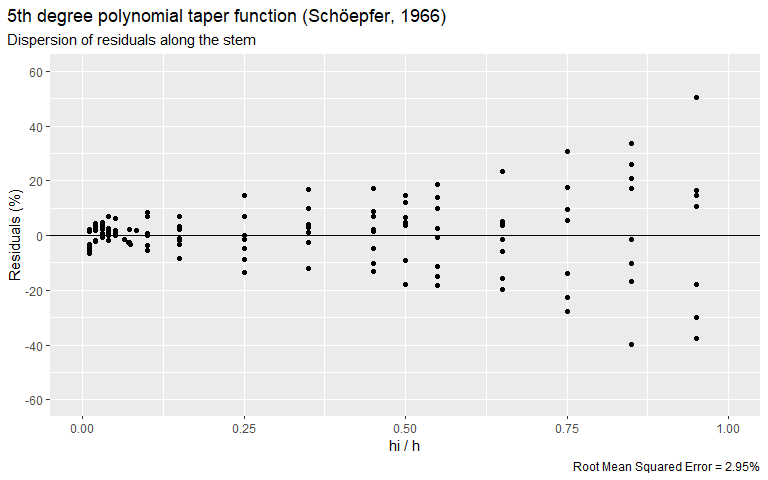The 5th degree polynomial is a fixed-form taper function that represents the average shape of the stem profiles used to fit the model. For this dataset, the Root Mean Square Error of this model was 3.01% and we can see that the residues are heteroskedastic. Let’s see if we can do better with the Bi model.Due to its non-linear nature, we will use the nlsLM function from the minpack.lm package to estimate the model parameters. library(minpack.lm) bi <- nlsLM(di ~ taper_bi(dbh, h, hih, b0, b1, b2, b3, b4, b5, b6), data=tree_scaling, start=list(b0=1.8,b1=-0.2,b2=-0.04,b3=-0.9,b4=-0.0006,b5=0.07,b6=-.14)) summary(bi) #> #> Formula: di ~ taper_bi(dbh, h, hih, b0, b1, b2, b3, b4, b5, b6) #> #> Parameters: #> Estimate Std. Error t value Pr(>|t|) #> b0 1.821275 0.455063 4.002 0.000105 *** #> b1 1.417831 0.287019 4.940 2.38e-06 *** #> b2 0.142028 0.035637 3.985 0.000112 *** #> b3 -1.082588 0.301635 -3.589 0.000470 *** #> b4 -0.004008 0.003088 -1.298 0.196525 #> b5 0.325254 0.053354 6.096 1.17e-08 *** #> b6 -0.735532 0.106476 -6.908 2.02e-10 *** #> --- #> Signif. codes: 0 '***' 0.001 '**' 0.01 '*' 0.05 '.' 0.1 ' ' 1 #> #> Residual standard error: 0.8063 on 129 degrees of freedom #> #> Number of iterations to convergence: 5 #> Achieved convergence tolerance: 1.49e-08 tree_scaling <- tree_scaling %>% mutate(di_bi = predict(bi)) bi_rmse <- tree_scaling %>% summarise(RMSE = sqrt(sum((di_bi-di)^2)/mean(di_bi))) %>% pull(RMSE) %>% round(2) ggplot(tree_scaling,aes(x=hih))+ geom_point(aes(y = (di_bi-di)/di_bi*100))+ geom_hline(aes(yintercept = 0))+ scale_y_continuous(limits=c(-60,60), breaks = seq(-100,100,20))+ scale_x_continuous(limits=c(0,1))+ labs(x = 'hi / h', y = 'Residuals (%)', title = 'Bi (2000) trigonometric variable-form taper function', subtitle = 'Dispersion of residuals along the stem', caption = paste0('Root Mean Squared Error = ', bi_rmse,'%'))+ theme(plot.title.position = 'plot')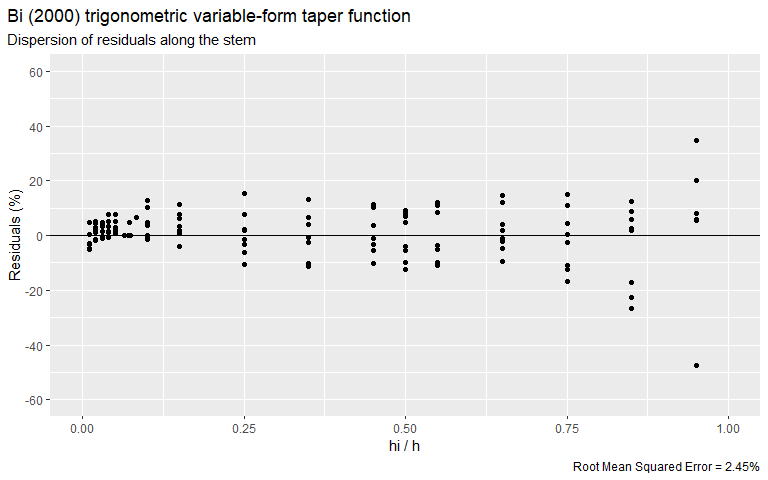The Bi model performed better than the polynomial function, based on the RMSE value. However, we still have heteroscedasticity in the residues. Let’s see what we get by adjusting the Kozak (2004) model. We will treat the p parameter of this model as one more to be estimated using the nlsLM function. kozak <- nlsLM(di ~ taper_kozak(dbh, h, hih, b0, b1, b2, b3, b4, b5, b6, b7, b8, p), start=list(b0=1.00,b1=.97,b2=.03,b3=.49,b4=- 0.87,b5=0.50,b6=3.88,b7=0.03,b8=-0.19, p = .1), data = tree_scaling, control = nls.lm.control(maxiter = 1000, maxfev = 2000) ) summary(kozak) #> #> Formula: di ~ taper_kozak(dbh, h, hih, b0, b1, b2, b3, b4, b5, b6, b7, #> b8, p) #> #> Parameters: #> Estimate Std. Error t value Pr(>|t|) #> b0 1.057e+00 5.338e-01 1.981 0.0498 * #> b1 1.088e+00 8.074e-02 13.471 < 2e-16 *** #> b2 1.120e-01 2.205e-01 0.508 0.6125 #> b3 3.404e-01 5.610e-02 6.067 1.41e-08 *** #> b4 -2.823e+00 4.948e-01 -5.706 7.83e-08 *** #> b5 9.832e-01 1.192e-01 8.251 1.79e-13 *** #> b6 1.197e+01 2.902e+00 4.123 6.73e-05 *** #> b7 1.082e-01 4.673e-02 2.316 0.0222 * #> b8 -4.930e-01 2.951e-01 -1.671 0.0972 . #> p 2.149e-18 6.523e-13 0.000 1.0000 #> --- #> Signif. codes: 0 '***' 0.001 '**' 0.01 '*' 0.05 '.' 0.1 ' ' 1 #> #> Residual standard error: 0.6276 on 126 degrees of freedom #> #> Number of iterations to convergence: 100 #> Achieved convergence tolerance: 1.49e-08 tree_scaling <- tree_scaling %>% mutate(di_kozak = predict(kozak)) kozak_rmse <- tree_scaling %>% summarise(RMSE = sqrt(sum((di_kozak-di)^2)/mean(di_kozak))) %>% pull(RMSE) %>% round(2) ggplot(tree_scaling, aes(x=hih))+ geom_point(aes(y = (di_kozak-di)/di_kozak*100))+ geom_hline(aes(yintercept = 0))+ scale_y_continuous(limits=c(-100,100), breaks = seq(-100,100,20))+ scale_x_continuous(limits=c(0,1))+ labs(x = 'hi / h', y = 'Residuals (%)', title = 'Kozak (2004) variable-form taper function', subtitle = 'Dispersion of residuals along the stem', caption = paste0('Root Mean Squared Error = ', kozak_rmse,'%'))+ theme(plot.title.position = 'plot')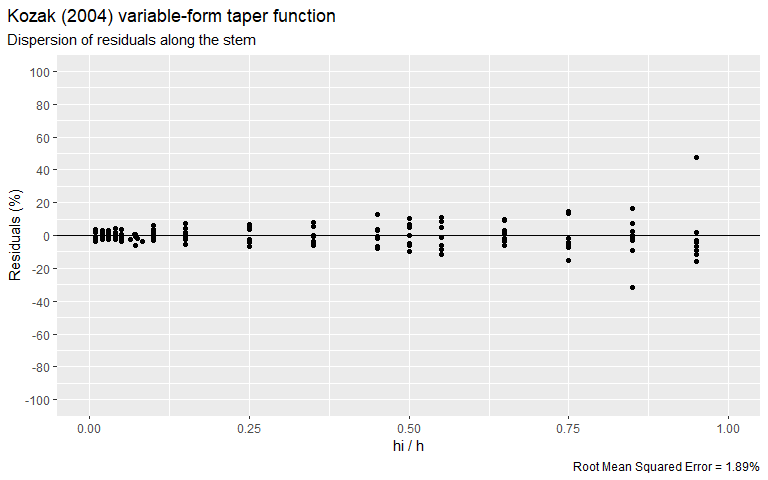By fitting the Kozak (2004) model, we obtained a lower RMSE and also managed to homogenize the dispersion of the residues. ### Using taper models In the previous section we adjusted the three models that have auxiliary functions implemented in the timbeR package. Now, let’s explore the functions that allow us to apply the fitted models in practice. The following table presents the auxiliary functions for the three supported models, grouped by usage. Usage 5° degree polynomial Bi (2002) Kozak (2004) Estimate the diameter at a given height poly5_di bi_di kozak_di Estimate the height at which a given diameter occurs poly5_hi bi_hi kozak_hi Estimate the total or partial volume of the stem poly5_vol bi_vol kozak_vol Estimate volume and quantity of logs per assortment poly5_logs bi_logs kozak_logs Visualize the simulation of log cutting along the stem poly5_logs_plot bi_logs_plot kozak_logs_plot Next, we will apply the functions described in the table using the models fitted in the previous section. For ease of understanding, let’s start by applying the functions to a single tree. Let’s define it’s total height and DBH measurements. dbh <- 25 h <- 20 All auxiliary functions have the argument coef, where a vector containing the fitted coefficients of the model must be declared. This vector can be accessed by using the base R function coef. For the Kozak (2004) model, we will separate the p parameter from the others. coef_poli <- coef(poli5) coef_bi <- coef(bi) coef_kozak <- coef(kozak)[-10] p_kozak <- coef(kozak) Now we can estimate the diameter (di) at a given height (hi). Let’s assume hi = 15 for this example. hi <- 15 poly5_di(dbh, h, hi, coef_poli) #>  9.224517 bi_di(dbh, h, hi, coef_bi) #>  8.559173 kozak_di(dbh, h, hi, coef_kozak, p = p_kozak) #>  8.92263 Note that there is some variation between the predictions of the models. We can better observe this effect by modeling the complete profile of our example tree. hi <- seq(0.1,h,.1) ggplot(mapping=aes(x=hi))+ geom_line(aes(y=poly5_di(dbh, h, hi, coef_poli), linetype = '5th degree polynomial'))+ geom_line(aes(y=bi_di(dbh, h, hi, coef_bi), linetype = 'Bi (2000)'))+ geom_line(aes(y=kozak_di(dbh, h, hi, coef_kozak, p_kozak), linetype = 'Kozak (2004)'))+ scale_linetype_manual(name = 'Fitted models', values = c('solid','dashed','dotted'))+ labs(x = 'hi (m)', y = 'Predicted di (cm)')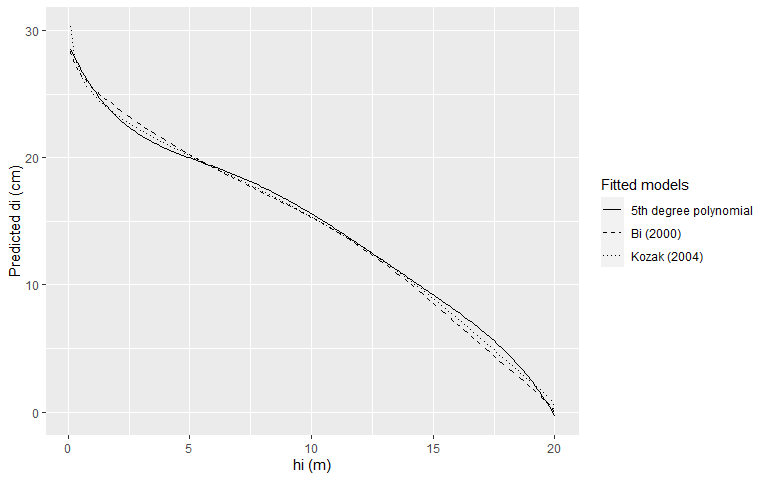For the prediction of the height at which a given diameter occurs the procedure is similar to the one presented above, but this time we must declare the argument di instead of hi, for the corresponding functions. di <- 10 poly5_hi(dbh, h, di, coef_poli) #>  14.40821 bi_hi(dbh, h, di, coef_bi) #>  14.09805 kozak_hi(dbh, h, di, coef_kozak, p_kozak) #>  14.2817 For this example the application of the three models resulted in very similar predictions. The functions for estimating total and partial volumes are similar to those presented so far, with some additional arguments. The following procedures calculate the volume of the entire stem. poly5_vol(dbh, h, coef_poli) #>  0.414718 bi_vol(dbh, h, coef_bi) #>  0.4128356 kozak_vol(dbh, h, coef_kozak, p_kozak) #>  0.413102 We can also estimate partial volumes by declaring the initial height h0 and the final height hi. hi = 15 h0 = .2 poly5_vol(dbh, h, coef_poli, hi, h0) #>  0.3884416 bi_vol(dbh, h, coef_bi, hi, h0) #>  0.3901346 kozak_vol(dbh, h, coef_kozak, p_kozak, hi, h0) #>  0.3863585 Finally, we will see how the three models estimate the volume and quantity of logs from different wood products. We start by defining the assortments. The assortment table must contain five columns, in order: the product name, the log diameter at the small end (cm), the minimum length (m), the maximum length (m), and the loss resulting from cutting each log (cm). Let’s transcribe the following table into a data.frame. A point of attention is that the wood products must be ordered in the data.frame from the most valuable to the least valuable, in order to give preference to the products of highest commercial value. Name SED MINLENGTH MAXLENGTH LOSS > 15 15 2.65 2.65 5 4-15 4 2 4.2 5 assortments <- data.frame( NAME = c('> 15','4-15'), SED = c(15,4), MINLENGTH = c(2.65,2), MAXLENGTH = c(2.65,4.2), LOSS = c(5,5) ) We can now estimate volume and quantity of wood products in a tree stem. poly5_logs(dbh, h, coef_poli, assortments) #>$volumes
#> # A tibble: 1 x 2
#>   > 15 4-15
#>    <dbl>  <dbl>
#> 1  0.293  0.111
#>
#> $logs #> # A tibble: 1 x 2 #> > 15 4-15 #> <dbl> <dbl> #> 1 3 2 bi_logs(dbh, h, coef_bi, assortments) #>$volumes
#> # A tibble: 1 x 2
#>   > 15 4-15
#>    <dbl>  <dbl>
#> 1  0.299  0.107
#>
#> $logs #> # A tibble: 1 x 2 #> > 15 4-15 #> <dbl> <dbl> #> 1 3 2 kozak_logs(dbh, h, coef_kozak, p_kozak, assortments) #>$volumes
#> # A tibble: 1 x 2
#>   > 15 4-15
#>    <dbl>  <dbl>
#> 1  0.296  0.109
#>
#> $logs #> # A tibble: 1 x 2 #> > 15 4-15 #> <dbl> <dbl> #> 1 3 2 There are several additional arguments in the log volume/quantity estimation functions that change the way the calculations are performed. It is highly recommended that you read the function’s help to understand all its functionality. An additional feature of the timbeR package is the possibility to visualize how the processing of trees is performed by the logs estimation functions. The arguments of these functions are practically the same arguments of the functions presented above. poly5_logs_plot(dbh, h, coef_poli, assortments)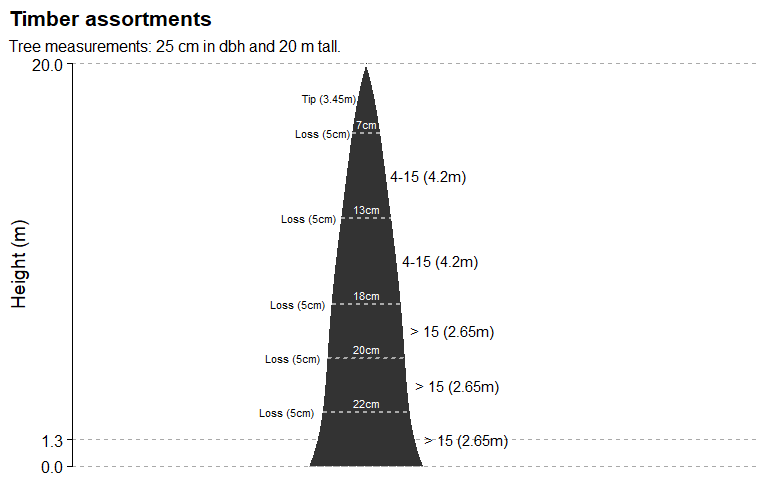bi_logs_plot(dbh, h, coef_bi, assortments)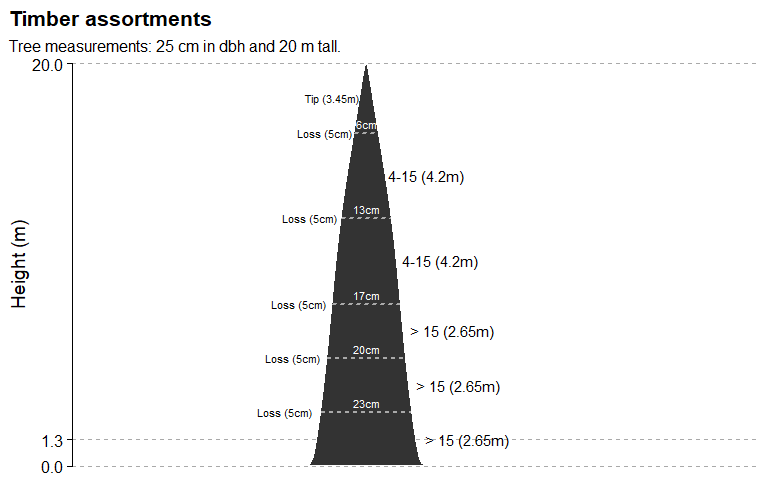kozak_logs_plot(dbh, h, coef_kozak, p_kozak, assortments)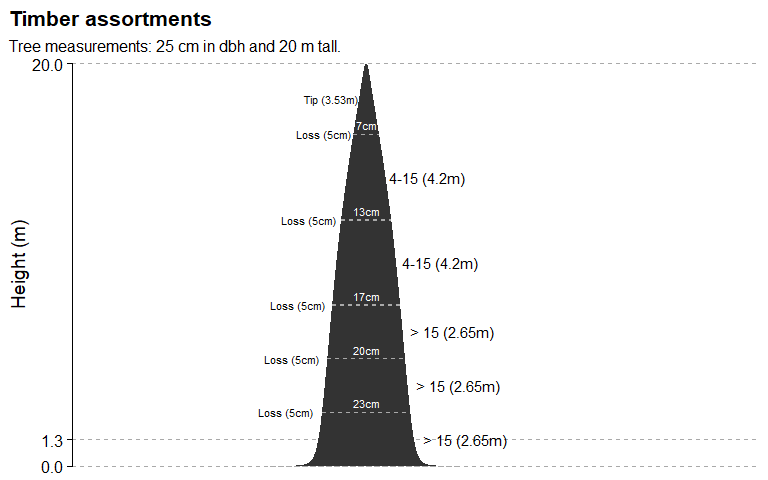### Using timbeR functions at forest inventory scale Log estimation functions are performed one tree at a time. Applying these functions to multiple trees can be performed in different ways. Below are some examples using the base R function mapply and using pmap function from purrr package. # Using mapply tree_data <- data.frame(dbh = c(18.3, 23.7, 27.2, 24.5, 20, 19.7), h = c(18, 24, 28, 24, 18.5, 19.2)) assortment_vol <- mapply( poly5_logs, dbh = tree_data$dbh,
h = tree_data\$h,
SIMPLIFY = T,
MoreArgs = list(
coef = coef_poli,
assortments = assortments,
stump_height = 0.2,
total_volume = T,
only_vol = T
)
) %>%
t()

assortment_vol
#>      > 15       4-15       Total
#> [1,] 0.06525096 0.124656   0.1999943
#> [2,] 0.3303831  0.09825193 0.4472505
#> [3,] 0.5307287  0.1305737  0.687288
#> [4,] 0.3530639  0.1051031  0.4779542
#> [5,] 0.1335897  0.09999402 0.2455131
#> [6,] 0.1310012  0.1044527  0.247216

# Bindind tree_data and volumes output

library(tidyr)

cbind(tree_data, assortment_vol) %>%
unnest()
#> # A tibble: 6 x 5
#>     dbh     h > 15 4-15 Total
#>   <dbl> <dbl>  <dbl>  <dbl> <dbl>
#> 1  18.3  18   0.0653 0.125  0.200
#> 2  23.7  24   0.330  0.0983 0.447
#> 3  27.2  28   0.531  0.131  0.687
#> 4  24.5  24   0.353  0.105  0.478
#> 5  20    18.5 0.134  0.100  0.246
#> 6  19.7  19.2 0.131  0.104  0.247
# Using pmap

library(purrr)

tree_data %>%
mutate(coef = list(coef_poli),
assortments = list(assortments),
stump_height = 0.2,
total_volume = T,
only_vol = T) %>%
mutate(assortment_vol = pmap(.,poly5_logs)) %>%
select(dbh, h, assortment_vol) %>%
unnest(assortment_vol)
#> # A tibble: 6 x 5
#>     dbh     h > 15 4-15 Total
#>   <dbl> <dbl>  <dbl>  <dbl> <dbl>
#> 1  18.3  18   0.0653 0.125  0.200
#> 2  23.7  24   0.330  0.0983 0.447
#> 3  27.2  28   0.531  0.131  0.687
#> 4  24.5  24   0.353  0.105  0.478
#> 5  20    18.5 0.134  0.100  0.246
#> 6  19.7  19.2 0.131  0.104  0.247